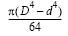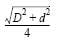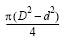Area Moment of Inertia Section Properties Tube/Pipe Calculator

Area Moment of Inertia Section Properties Tube/Pipe Calculator

Area Moment of Inertia Section Properties of Tube/Pipe Feature Calculator and Equations. This engineering calculator will determine the section modulus for the given cross-section. This engineering data is often used in the design of structural beams or structural flexural members.

 Description Equation Area Moment of Inertia Section Properties = ISection Modulus = Z = I/yRadius of GyrationA = areay = Distance to neutral axis D/2Area Moment of Inertia Section Properties Tube/Pipe Calculator Variables Inputs: Inch (in.) Metric (mm) "D" (O.D.) = "d" (I.D.) = Properties: Area Moment of Inertia Section Properties (Units4) = Section Modulus (Units3) = Radius of Gyration (Units) = Extreme Point (Units) = Area (Units2) =Membership Register | LoginHomeEngineering Book StoreEngineering ForumExcel App. DownloadsOnline Books & ManualsEngineering NewsEngineering VideosEngineering CalculatorsEngineering ToolboxGD&T Training Geometric Dimensioning TolerancingDFM DFA TrainingTraining Online EngineeringAdvertising CenterCopyright Notice